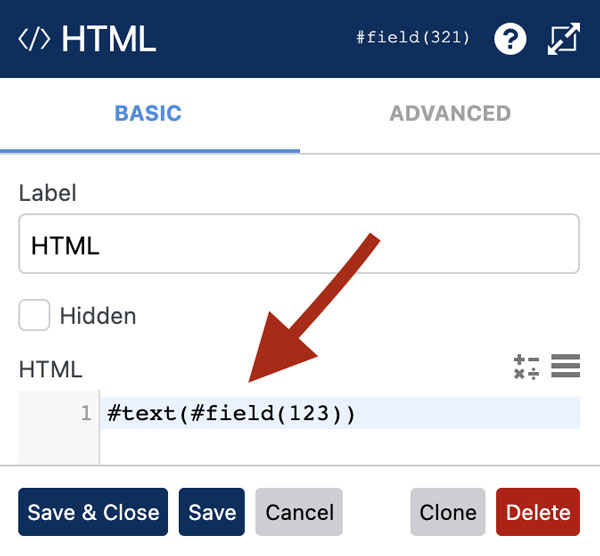# Create a Form SummaryPRO

On a long form you may wish to add a summary / review of the fields the user has completed. In this tutorial we explain how to create a  summary using the `#text` variable. It also shows how you can create a table containing the contents of a repeatable section by using the `#section_rows_start(section_id)` and `#section_rows_end` variables.

### How It Works

In the example above, an HTML field is used to output a summary of the complete fields. The `#field` variable is used to insert the value of a field in the HTML field. This is wrapped in the `#text` variable which tells WS Form to keep the field value updated in the HTML field whenever the value changes.

An example of this syntax is: `#text(#field(123))`

An example of this added to an HTML field is show below:A working example of this is shown below:

The `#text` field can also be used in text editor, message or even the default value of fields such as text, phone or email.

You can combine these variables with regular HTML code to style and format the output as you wish.

### Conditionally Showing Field Values

Sometimes you may only wish to show a field value if it contains a value. To do this, you can use the `#if(condition)` and `#endif` variables.

#### Example

```#if(#field(1))
<p><strong>#field_label(1)</strong><br />#text(#field(1))</p>
#endif

#if(#field(2))
<p><strong>#field_label(2)</strong><br />#text(#field(2))</p>
#endif
```

In this example, WS Form checks to see if the field with ID 1 has content. If it does, it shows the content between the `#if(#field(1))` and `#endif` variables. This functionality is then repeated for the field with ID 2.

### Repeatable Sections

You can also create a summary of fields contained within a repeatable section.

Example code to do this is shown below:

```<table>

<tr>
<th width="20">#</th>
<th>#field_label(1)</th>
<th>#field_label(2)</th>
</tr>

<tbody>

#section_rows_start(10)
<tr>
<th>#section_row_number</th>
<td>#text(#field(1))</td>
<td>#text(#field(2))</td>
</tr>
#section_rows_end

</tbody>

</table>```

In this example, we are outputting a simple HTML table. The rows in the table are wrapped in `#section_rows_start(section_id)` and `#section_rows_end`. WS Form then repeats the HTML contained within for each row in the repeatable section that has an ID of 10. The variable `#section_row_number` outputs the row number.